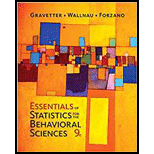Chapter 2.1, Problem 1LCEssentials of Statistics for The B...

9th Edition
Frederick J Gravetter + 2 others
ISBN: 9781337098120

Solutions

Chapter
SectionEssentials of Statistics for The B...

9th Edition
Frederick J Gravetter + 2 others
ISBN: 9781337098120
Textbook Problem

If the following scores are placed in a frequency distribution table, then what is the frequency value corresponding to X = 2? Scores: 2, 3, 1, 1, 3, 3, 2, 4, 3, 1 a. 1 b. 2 c. 3 d. 4

To determine

To find: The frequency value corresponding to X=2 .

Explanation

Reason for correct answer:

The Scores (X) are in discrete variables given as:

X2311332431

Frequency distribution is showing the frequency of an event. In other words, it contains count of occurrences of individual event. X repeated two times in the distribution table so option (b) 2 is correct.

Software procedure:

Step-by-step procedure to obtain the frequency distribution table using the SPSS software:

• Enter the data under X column.
• Go to Analyze > select Descriptive statistics > click on Frequencies.
• Highlight the column label (X) in the left box then click arrow to receive it into variable box

Still sussing out bartleby?

Check out a sample textbook solution.

See a sample solution

The Solution to Your Study Problems

Bartleby provides explanations to thousands of textbook problems written by our experts, many with advanced degrees!

Get Started

Evaluate the integral. 03/2sinxdx

Single Variable Calculus: Early Transcendentals, Volume I